# How to recover the Borrow Bit

In a similar way as the carry bit for addition it is possible to recover the borrow bit for substraction, just based on the highest bits of three numbers that we deal with during the operation.

With this program, a subtraction operation of an 8-bit CPU can be simulated exhaustively

 #!/usr/bin/perl

 my $x,$x, $bi; my %tab = (); for ($bi = 0; $bi <= 1;$bi++) {     for ($x = 0;$x < 256; $x++) { for ($y = 0; $y < 256;$y++) {             my $zz =$x - $y -$bi;             my $b =$zz < 0 ? 1 : 0;             my $c = 1 -$b;             my $z = ($zz + 256) & 0xff;             my $xs =$x >> 7;             my $ys =$y >> 7;             my $zs =$z >> 7;             my $key = "$xs:$ys:$zs";             $tab{$key} //= $b; my$bb = $tab{$key};             if ($bb !=$b) {                 print "b=$b bb=$bb c=$c xs=$xs ys=$ys zs=$zs x=$x y=$y z=$z zz=$zz bi=$bi\n"; } } } }  for my$key (sort keys %tab) {     $key =~ m/(\d+):(\d+):(\d+)/;$xs=$1;$ys=$2;$zs=$3;$b =$tab{$key};     $c = 1 -$b;     $bb =$xs & $ys &$zs | !$xs & ($ys | $zs); print "b=$b bb=$bb c=$c xs=$xs ys=$ys zs=$zs\n"; }  This gives an idea, what is happening. But in real life, probably a 64bit-CPU is used, but the concepts would work with longer or shorter CPU words the same way. So we subtract two unsigned 64-bit integers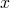and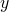and an incoming borrow bit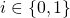to a result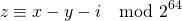with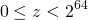using the typical „long long“ of C. We assume that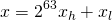where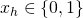and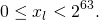In the same way we assumeand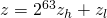with the same kind of conditions for,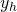,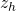or,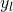,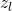, respectively. Now we have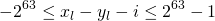and we can see thatfor some. And we have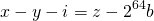where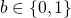is the borrow bit. When combining we get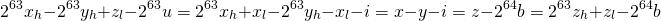When looking just at the highest visible bit and the borrow bit, this boils down to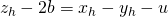This leaves us with eight cases to observe for the combination of,and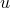: x_hy_huz_hb 00000 00111 01011 01101 10010 10100 11000 11111 Or we can check all eight cases and find that we always haveSo the result does not depend onanymore, allowing to calculate the borrow bit by temporarily casting,and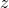to (signed long long) and using their sign. We can express this as „use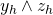if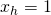and use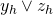if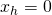„. The incoming borrow bitdoes not change this, it just allows for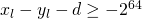, which is sufficient for making the previous calculations work. The basic operations add, adc, sub, sbb, mul, xdiv (div is not available) have been implemented in this library for C. Feel free to use it according to the license (GPL). Addition and subtraction could be implemented in a similar way in Java, with the weirdness of declaring signed longs and using them as unsigned. For multiplication and division, native code would be needed, because Java lacks 128bit-integers. So the C-implementation is cleaner. # Exceptions to implement Program Logic Sometimes it is conveniant to use exceptions for implementing the regular program logic. Assuming we want to find some data and then process them. When no data is found, this step can be skipped, because there is nothing to do. So we could program something like this:  public Data readData(Param param) { Data data = db.read(param); if (data.isEmpty()) { throw new NotFoundException("nothing found"); } return data; }   public ProcessedData doWork(Param param) { try { Data input = readData(param); .... .... .... ProcessedData result = .... return result; } catch (NotFoundException nfex) { return ProcessedData.empty(); } }  And some other exceptions could also be handled in a similar way. Of course some people say, that this is not good and an abuse of exceptions. But sometimes it is tempting. So is this bad? And if so, why? Let’s find out. This is some kind of weird obfuscation of the control flow, because throwing and catching of exceptions can be far apart and it can become quite unclear, from where in the stack which exceptions can be thrown. So there are good reasons to recommend using exceptions only for what they are meant for by their name. The Goto has never made it into Java and we are discouraged from using it in many other languages, like C. But languages like Java, C, Perl, Ruby and some others provide quite rich control flow relying neither on goto nor on exceptions by using „return“ anywhere in a function or method or subroutine, leaving loops with „break“ or „last“ or going to the next iteration with „next“ or „continue“. Perl and Java even allow to specify which of nested loops to leave with break or last. These mechanisms are very powerful and there is no urgent need to add exceptions or even gotos just to support the control flow. Once moving to newer languages like Scala much of this is gone or at least strongly discouraged in a purely functional programming style. This makes programming Scala harder, and comes with benefits that might be worth the extra effort. But in Java these functional purists have not become very strong yet, so using „break“, „continue“, „return“ etc. is still ok and quite powerful. In Java there is another very major problem with exceptions. Many, if not most Java programs run in a framework or container like Spring, EJB/JEE, JBoss Fuse, for example. Now a piece of software becomes a software component, that can interact with other components through the framework. And exceptions are noticed by the framework. In many cases they have the effect that an ongoing transaction is marked as „rollback only“. So the whole processing continues normally, and when all the code from the components is finally done, the framework performs a rollback instead of a commit. As long as exceptions are only used for handling errors or unsual situations, in which cases the rollback is probably the way to go anyway, everything is fine. But if we for example look up something and base the further processing on the outcome of this, then a NotFoundException will result in very counter intuitive behavior. So the original rule of not abusing exceptions is actually not such a bad idea. # How to use$ in Articles using WP QuickLaTeX

I use WP QuickLaTeX by Pavel Holoborodko in some of my articles to include mathematical formulas.
Now it can be an issue that the „$“-sign, that marks the beginning of a formula, is used as dollar sign. This can be achieved by using [latexpage] in the beginning of the page. Sometimes it is desirable, to apply this only to part of the page. This is achieved using [latexregion]. The following $ this is a dollar sign [latexregion] And this is a full line formular $$a^2+b^2=c^2$$ And this is an inline formula $a^2+b^2=c^2$. With more stuff... [/latexregion] And here dollar signs  are dollar signs again. 
results in:

\$ this is a dollar sign

And this is a full line formularAnd this is an inline formula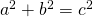. With more stuff…

And here dollar signs  are dollar signs again.

And yes, to show the
 [latexregion] ... [/latexregion] 
above, I had to actually type
 &#91;latexregion&#93; ... &#91;/latexregion&#93; 
Let’s stop the recursion here…